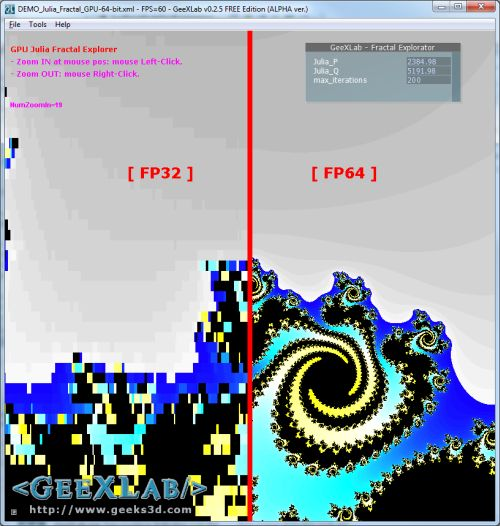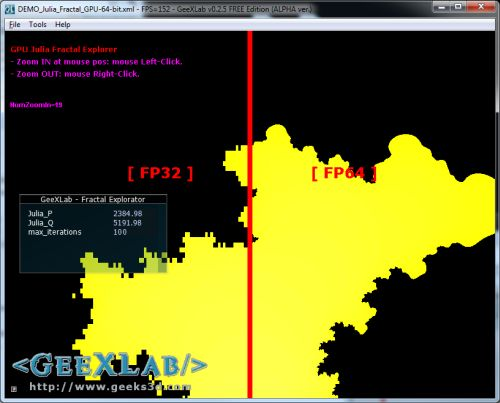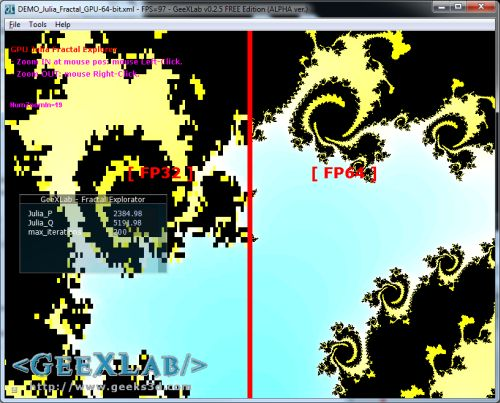# (Tested) Double Precision FP64 in GLSL: Julia Explorer DemoWant to see double precision (FP64) in GLSL in action? Here is a small GeeXLab demo that renders a Julia fractal in single precision floating point (fp32) and in double precision floating point (fp64) at the same time.

The Mandelbrot / Julia fractal is a nice example for showing the advantage of fp64 in shaders. On the left side, the rendering is done with fp32 and on the right side, fp64 variables are used. An unique GLSL shader is used for both fp32 and fp64 rendering. More information on the Mandelbrot fractal can be found here: The Mandelbrot Set: Colors of Infinity.

Unzip the archive somewhere, and launch the file called DEMO_Julia_Fractal_GPU-64-bit.exe. That’s all.

If your graphics card supports fp64 in OpenGL shaders (read if your card supports the GL_ARB_gpu_shader_fp64 extension) you should see something like the image above, otherwise I don’t know 😀 I only tested with a GTX 460 and a HD 5870 and I didn’t add fp64 checks (see below for some details about Radeon HD 5870)…

Controls: use mouse left click to zoom in at the mouse position, and mouse right click to zoom out. After around 20 zoom in, you should see the limitation of fp32 calculations.

The source code is available in the DEMO_Julia_Fractal_GPU-64-bit.xml file.

The tweak bar allows you to change the number of iterations. By default there are 100 iterations per pixel. Increasing the number of iterations offers a better precision but drops the FPS:Julia set with 100 iterations, 150FPS (GTX 460)Julia set with 200 iterations, 97FPS (GTX 460)

I tested this demo on a GeForce GTX 460 (MSI N460GTX Cyclone) + R260.63 without problem. I also tested the demo on a Radeon HD 5870 + Catalyst 10.9 and the first version of the demo didn’t work on AMD’s card. After a quick investigation, it turned out that on Radeon, the fp64 uniforms were not transmitted to the shader. Maybe a bug in Catalyst 10.9, I don’t know yet. The workaround I found is simple: cast fp32 uniform variables into fp64. That works fine for this demo but this trick is not the solution because you lose precision when you cast fp32 to fp64.

Here is the complete source code the Julia GLSL shader (available in the demo source code):

```[Vertex_Shader]
#version 400 compatibility
void main(void)
{
gl_Position = ftransform();
gl_TexCoord = gl_MultiTexCoord0;
}

#version 400 compatibility

uniform double center_x_d;
uniform double center_y_d;
uniform float center_x_f;
uniform float center_y_f;

uniform int max_iterations;
uniform sampler1D color_palette;

uniform dvec2 c_d;
uniform vec2 c_f;
uniform float max_size_f;
uniform double max_size_d;

int mandel_d(dvec2 p)
{
dvec2 z = dvec2(0.0);
int iterations = 0;
while(iterations < max_iterations)
{
dvec2 z2 = z*z;
if (z2.x + z2.y > 4.0)
break;
z = dvec2(z2.x - z2.y, 2.0 * z.x * z.y) + p;
iterations++;
}
return iterations;
}
int julia_d(dvec2 p, dvec2 ab)
{
dvec2 z = p;
int iterations = 0;
while(iterations < max_iterations)
{
dvec2 z2 = z*z;
if (z2.x + z2.y > 4.0)
break;
z = dvec2(z2.x - z2.y, 2.0 * z.x * z.y) + ab;
iterations++;
}
return iterations;
}
int mandel_f(vec2 p)
{
vec2 z = vec2(0.0);
int iterations = 0;
while(iterations < max_iterations)
{
vec2 z2 = z*z;
if (z2.x + z2.y > 4.0)
break;
z = vec2(z2.x - z2.y, 2.0 * z.x * z.y) + p;
iterations++;
}
return iterations;
}
int julia_f(vec2 p, vec2 ab)
{
vec2 z = p;
int iterations = 0;
while(iterations < max_iterations)
{
vec2 z2 = z*z;
if (z2.x + z2.y > 4.0)
break;
z = vec2(z2.x - z2.y, 2.0 * z.x * z.y) + ab;
iterations++;
}
return iterations;
}
void main (void)
{
int num_iterations = 0;
vec4 final_color = vec4(0.0, 0.0, 0.0, 1.0);

if (gl_TexCoord.x < 0.495)
{
vec2 position  = vec2(gl_TexCoord.xy - 0.5) * max_size_f;
float c_real = position.x + center_x_f;
float c_imag = position.y + center_y_f;
//num_iterations = mandel_f(vec2(c_real, c_imag));
num_iterations = julia_f(vec2(c_real, c_imag), vec2(c_f.x, c_f.y));
final_color = texture1D(color_palette, \
float(num_iterations == max_iterations ? 0 : num_iterations) / max_iterations);
}

/*
// Works on NVIDIA only (on ATI: maybe a bug in the fp64 uniform \
functions in OpenGL API).
else if (gl_TexCoord.x > 0.505)
{
dvec2 position  = dvec2(gl_TexCoord.xy - 0.5) * max_size_d;
double c_real = position.x + center_x_d;
double c_imag = position.y + center_y_d;
//num_iterations = mandel_d(dvec2(c_real, c_imag));
num_iterations = julia_d(dvec2(c_real, c_imag), dvec2(c_d.x, c_d.y));
final_color = texture1D(color_palette, \
float(num_iterations == max_iterations ? 0 : num_iterations) / max_iterations);
}
*/

// Works on NVIDIA and ATI.
else if (gl_TexCoord.x > 0.505)
{
dvec2 position  = dvec2(gl_TexCoord.xy - 0.5) * double(max_size_f);
double c_real = position.x + double(center_x_f);
double c_imag = position.y + double(center_y_f);
//num_iterations = mandel_d(dvec2(c_real, c_imag));
num_iterations = julia_d(dvec2(c_real, c_imag), dvec2(c_d.x, c_d.y));
final_color = texture1D(color_palette, \
float(num_iterations == max_iterations ? 0 : num_iterations) / max_iterations);
}
else
{
final_color = vec4(1.0, 0.0, 0.0, 1.0);
}
gl_FragColor = final_color;
}
```

## 6 thoughts on “(Tested) Double Precision FP64 in GLSL: Julia Explorer Demo”

1.Korvin77

“has stopped working” on my Radeon 4850 & Win7 x64. finally I see why I should buy new DX11 hardware 😀

2.JeGX Post Author

Thanks for the feedback. I will update the demo with, at least, the test of GL_ARB_gpu_shader_fp64 support!

3.Jose

Mine crashes too, GTX260 on Win7 x64

4.Jose

Oh i didn’t even see it requires a newer GPU, my bad sorry.

5.Cat

On a 5770 and Cat 10.9, the FP64 rendering is completely wrong even on the initial zoom level.